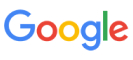New update is available. Click here to update.
Topics

# Cakes

Easy0/40
Average time to solve is 15m
1 upvote## Problem statement

You have 'N' cakes of different names. You want to choose exactly 3 cakes out of these 'N' cakes. The name of the ith cake is 'S[i]' (0 <= i < 'N'). The three chosen cakes should follow the following conditions.

``````The name of the cake should start with 'c', 'a', 'k', 'e', or 's'.
No two cakes should begin with the same letter.
``````

Return the number of ways to choose three cakes irrespective of the order.

Note : Assume 0-based indexing.

For example:
``````Let 'N' = 4, S = ["choco", "apple", "kesar", "strawberry"].
There exist 4 different ways to choose three cakes following all the required conditions i.e. {"choco", "apple", "kesar"}, {"choco", "apple", "strawberry"}, {"choco", "strawberry", "kesar"}, {"strawberry", "apple", "kesar"}.
``````
Detailed explanation ( Input/output format, Notes, Images )
Constraints:-
``````1 <= 'T' <= 10
1 <= 'N' <= 10^4
0 <= |S[i]| <= 10
All the names of cakes are distinct and consist of lowercase letters.

Time Limit: 1 sec
``````
Sample Input 1:-
``````2
4
apple kesar strawberry peach
2
apple kesar
``````
Sample Output 1:-
``````1
0
``````
Explanation of sample input 1:-
``````'N' = 4, S = ["apple", "kesar", "strawberry", "peach"].
There exists only one way to choose three cakes following all the required conditions i.e. {"strawberry", "apple", "kesar"}. So, the answer is 1.

Second test case:-
'N' = 2, S = ["apple", "kesar"]. As 'N' is less than 3, So there is no way to choose the three cakes. Hence, the answer is 0.
``````
Sample Input 2:-
``````2
5
apple kesar strawberry peach almond
1
apple
``````
Sample Output 2:-
``````2
0
``````Console